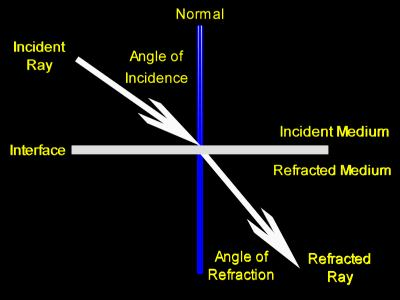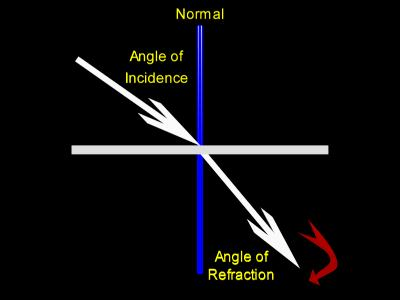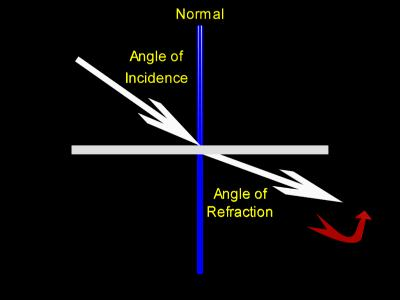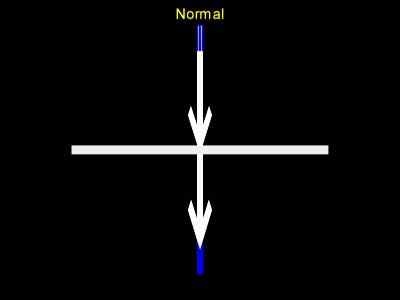# Ray Optics, Light Refraction

In the ray traced image above several clear glass spheres refract light from varied positions within a checkerboard tiled room.

Refraction occurs when a light ray changes mediums.

Light traveling from air and going into water would be an example of light changing mediums.

The speed of light changes when it changes mediums. In almost every case the direction of the light ray changes also.

An aside:

In relativity physics we say that the speed of light is always measured to be the same value regardless of the frame of reference, and this speed is, therefore, constant. In that context, though, we are speaking of the speed of light in free space traveling through a perfect vacuum. Here, discussing ray optics refraction, we are dealing with light traveling through a medium, like air or water, and judging its speed through that medium through a frame of reference at rest with the medium -- the lab.

In the picture above light rays coming from the walls and floor change direction as they go from air into the glass spheres, and they change direction again when they exit the glass spheres and renter air. This causes the checkerboard patterns to be distorted. Anyone who has ever looked through any type of curved piece of glass has seen a similar phenomena.At first we will look at a simple refraction, one that happens at a flat boundary. To the left is a picture of such a situation. Here a single ray of light strikes a boundary between two mediums and is refracted.

Note that the angle of refraction is smaller than the angle of incidence.

Here are descriptions for the terms in the above diagram:

• The ray of light which travels through the incident, or first, medium and strikes the boundary, or interface, is called the incident ray.
• The ray of light which travels into the refracted, or second, medium and leaves the interface is called the reflected ray.
• A line perpendicular to the surface is imagined at the point of refraction. This line is called a normal. In this context the word normal means perpendicular. In the above diagram the normal is colored blue.
• The angle between the incident ray and the normal is called the angle of incidence, or the incident angle.
• The angle between the refracted ray and the normal is called the angle of refraction, or the refracted angle.

The above picture demonstrates the general behavior of a light ray as it travels from air into some transparent medium such as water or glass.

We will often describe the light beam as bending toward the normal or away from the normal. The following picture, much like the one directly above, shows light bending toward the normal. Again, this is the general behavior for light going from air into water or glass.Here light bends toward the normal. The red arrow is meant to show what we mean by the phrase 'bending toward the normal.'

Notice that in these conditions the angle of refraction is smaller than the angle of incidence.

This next picture shows light bending away from the normal. This would be the general behavior for light going from water or glass into air.Here light bends away from the normal. The red arrow is meant to show what we mean by the phrase 'bending away from the normal.'

Notice that in these conditions the angle of refraction is larger than the angle of incidence.

The situations where light bends away from the normal can become more complicated than those where light bends toward the normal. This is because the angle of incidence can become large enough so that the angle of refraction, being even larger, can reach 90 degrees. This eliminates the possibility that the ray will enter the refracted medium. These conditions demonstrate what is known as a critical angle.Almost all of the time when light changes mediums, it changes direction. However, if it strikes the interface with an angle of incidence of 0 degrees, then no bending will occur. The picture at the left shows this

Also, there are a few conditions where light travels from one medium into another and does not bend for any angle of incidence. This happens when the two mediums are different substances, but have the same optical density. This situation will be covered in the explanation of Snell's law, which has its own section.

Custom Search